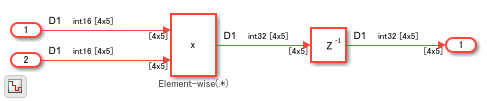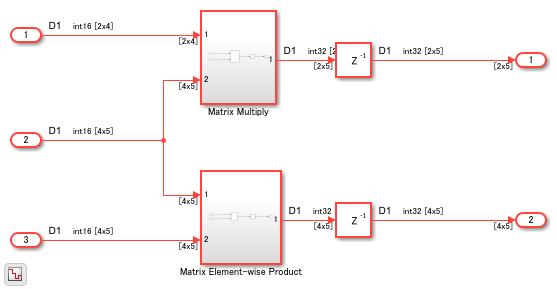## Design Considerations for Matrices and Vectors

These guidelines recommend how you can use matrix and vector signals when modeling your design for HDL code generation. Each guideline has a severity level that indicates the level of compliance requirements. To learn more, see HDL Modeling Guidelines Severity Levels.

### Modeling Requirements for Matrices

Guideline ID

`1.3.1`

Severity

Mandatory

Description

HDL Coder™ does not support matrix data types at the DUT interfaces. Before the 2-D matrix signals enter the DUT Subsystem, convert the signals to 1-D vectors by using a Reshape block. Inside the DUT Subsystem, you can convert the vectors back to matrices by using Reshape blocks, and then perform matrix computations. After performing computations, you must convert the matrices back to vector signals at the DUT output interface. Outside the DUT interface, you can convert the vector signals back to matrices.

Modeling Considerations

• When you use the Reshape block to convert vectors to 2D matrices, make sure that you specify the right Output dimensions.

• When you use the Product block, use the right Multiplication mode. By using this mode, you can perform either matrix multiplication or element-wise multiplication. The multiplied output can have different dimensions depending on the Multiplication mode.

• When you use the Product block to perform matrix multiplication, place the Matrix Multiply block inside a Subsystem block. When you generate code and open the generated model, you see that HDL Coder expands the matrix multiplication to multiple Product and Add blocks. Placing the Matrix Multiply block inside a subsystem makes the generated model easier to understand. In addition, make sure that you do not provide more than two inputs to the Matrix Multiply block.

• When you extract matrix data, use Selector and Assignment blocks. Make sure that you do not use fixed-point data types for the index input ports for the blocks.

Example

This example shows how to use matrix types in HDL Coder™. Open this model `hdlcoder_matrix_multiply`. The model contains a Reshape block that converts the matrix input to a 1-D vector at the DUT Subsystem interface.

```open_system('hdlcoder_matrix_multiply') set_param('hdlcoder_matrix_multiply', 'SimulationCommand', 'update') sim('hdlcoder_matrix_multiply') ```If you open the DUT Subsystem, you see two subsystems. The Reshape blocks convert the 1-D array back to the 2-by-2 matrices for input to the subsystems. One subsystem uses a Matrix Multiply block and the other subsystem performs element-wise multiplication. The output result is converted back to vectors.

```open_system('hdlcoder_matrix_multiply/DUT') ```If you generate HDL code for the DUT Subsystem and open the generated model, you see how the multiplication operation is performed.

### Avoid Generating Ascending Bit Order in HDL Code From Vector Signals

`1.3.2`

#### Severity

Strongly Recommended

#### Description

In MATLAB®, the default bit ordering for arrays is ascending. The generated VHDL code in such cases uses a declaration of ```std_logic_vector (0 to n)```. This signal declaration generates warnings by violating certain HDL coding standard rules. These are some scenarios:

Ascending Bit Order Scenarios

ScenarioProblem ExampleWorkaround

Delay block with a Delay length greater than `1`.

This example illustrates the generated code for a Delay block with a Delay length of `5`.

```ENTITY Subsystem1 IS PORT( clk : IN std_logic; reset : IN std_logic; enb : IN std_logic; In1 : IN std_logic; -- ufix1 Out1 : OUT std_logic -- ufix1 ); END Subsystem1; ARCHITECTURE rtl OF Subsystem1 IS -- Signals SIGNAL Delay_reg : std_logic_vector(0 TO 4); -- ufix1  SIGNAL Delay_out1 : std_logic; -- ufix1 ```

Instead of using a Delay block with a Delay length of `5`, you can connect five Delay blocks that have a Delay length of `1` in series.

```ENTITY Subsystem1 IS PORT( clk : IN std_logic; reset : IN std_logic; enb : IN std_logic; In1 : IN std_logic; -- ufix1 Out1 : OUT std_logic -- ufix1 ); END Subsystem1; ARCHITECTURE rtl OF Subsystem1 IS -- Signals SIGNAL Delay_out1 : std_logic; -- ufix1 SIGNAL Delay1_out1 : std_logic; -- ufix1 SIGNAL Delay2_out1 : std_logic; -- ufix1 SIGNAL Delay3_out1 : std_logic; -- ufix1 SIGNAL Delay4_out1 : std_logic; -- ufix1 ```

Combining multiple input signals to a vector signal using the Mux block.

This example illustrates the generated code when you use a Mux block to combine `4` input signals.

```ENTITY Subsystem IS PORT( In1 : IN std_logic; -- ufix1 Out1 : OUT std_logic_vector(0 TO 3) -- ufix1  ); END Subsystem; ARCHITECTURE rtl OF Subsystem IS -- Signals SIGNAL Mux_out1 : std_logic_vector(0 TO 3); -- ufix1  ```

Use a Bit Concat block to combine the input signals. This example illustrates the generated code for this block by concatenating `4` input signals.

```ENTITY Subsystem IS PORT( In1 : IN std_logic; -- ufix1 Out1 : OUT std_logic_vector(3 DOWNTO 0) -- ufix4 ); END Subsystem; ARCHITECTURE rtl OF Subsystem IS -- Signals SIGNAL Bit_Concat_out1 : unsigned(3 DOWNTO 0); -- ufix4 ```

Using a Constant block to generate vector signals.

This example illustrates the generated code when you use a Constant block to generate a vector of `4` scalar boolean signals.

```ENTITY Subsystem2 IS PORT( Out1 : OUT std_logic_vector(0 TO 3) -- boolean  ); END Subsystem; ARCHITECTURE rtl OF Subsystem2 IS -- Signals SIGNAL Constant_out1 : std_logic_vector(0 TO 3); -- boolean  ```

Use a Demux block followed by a Bit Concat block after the Constant block. This example illustrates the generated code when you apply this modeling technique to the vector of `4` Constant block.

```ENTITY Subsystem2 IS PORT( Out1 : OUT std_logic_vector(3 DOWNTO 0) -- ufix4 ); END Subsystem2; ARCHITECTURE rtl OF Subsystem2 IS -- Signals SIGNAL Constant_out1 : std_logic_vector(0 TO 3); -- boolean  SIGNAL Constant_out1_0 : std_logic; SIGNAL Constant_out1_1 : std_logic; SIGNAL Constant_out1_2 : std_logic; SIGNAL Constant_out1_3 : std_logic; SIGNAL Bit_Concat_out1 : unsigned(3 DOWNTO 0); -- ufix4 ] ```